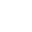• FAQ
• Contact/ Homework Answers / Physics / Question Five point charges, q1 = + q, q2 = +
Not my Question
Flag Content

# Question : Question Five point charges, q1 = + q, q2 = +Question

Five point charges, q1 = + q, q2 = + 2q, q3 = - 3q, q4 = - 4q, and q5 = - 5q, are placed in the vicinity of an insulating spherical shell with a charge + Q,                    distributed uniformly over its surface, as indicated in the figure.  Rank the point charges in order of decreasing magnitude of the force exerted on them by the sphere.

Five point charges, q1 = + q, q2 = + 2q, q3 = - 3q, q4 = - 4q, and q5 = - 5q, are placed in the vicinity of an insulating spherical shell with a charge + Q, distributed uniformly over its surface, as indicated in the figure. Rank the point charges in order of decreasing magnitude of the force exerted on them by the sphere.

## Solution 5 (1 Ratings )

Solved
Physics 9 Months Ago 2 Views